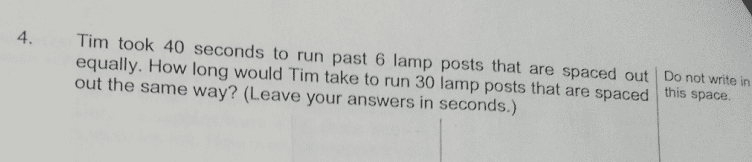# QuestionCan help?

I don’t understand why is the answer 232s.

L <> L <> L <> L <> L <> L

6 lamps (L)
5 intervals (<>)

Draw out the 6 lamp posts.  There should be 5 intervals between the lamp posts.

So to traverse 1 interval, Tim will need 40/5 = 8s.

For 30 lamp posts, there will be 29 intervals.

So Tim will need 29 x 8 = 232s

0 Replies 1 Like